Courses

# Mensuration Basics and 3-Dimensional Geometry Concepts Quant Notes | EduRev

## Banking Exams : Mensuration Basics and 3-Dimensional Geometry Concepts Quant Notes | EduRev

The document Mensuration Basics and 3-Dimensional Geometry Concepts Quant Notes | EduRev is a part of the Banking Exams Course Quantitative Aptitude (Quant).
All you need of Banking Exams at this link: Banking Exams

What is Mensuration?

A branch of mathematics that talks about the length, volume, or area of different geometric shapes is called Mensuration. These shapes exist in 2 dimensions or 3 dimensions.

CAT Mensuration Syllabus Topics & Formulas

 SOLID Total Surface Area Lateral/ Curved Surface area Volume Length of Leading Diagonal/ Slant Height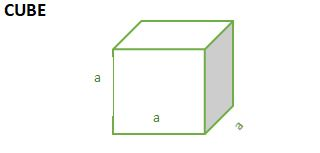6a2 4a2 a3 √3a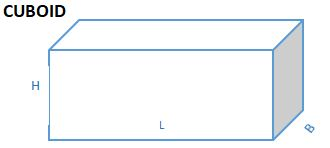2(LB+ BH+ HL) 2H (L + B) LBH √ (L2 + H2 + B2)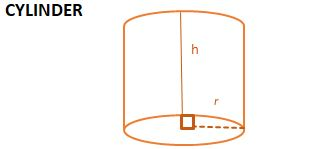2Πr (r + h) 2Πrh Πr2h No Slant height or diagonal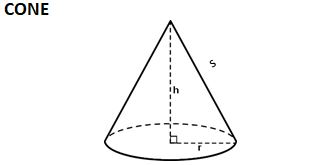Πr (r + l) Πrl ⅓Πr2h √(h2 + r2)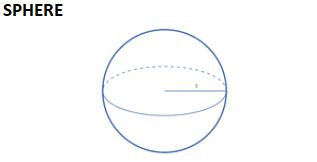4Πr2 4Πr2 4/3Πr3 No Slant height or diagonal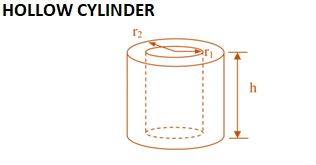2Π(r₁+r₂) (r₂-r₁+h) 2Πh(r₁+r₂) Πh(r₂²-r₁²) No Slant height or diagonal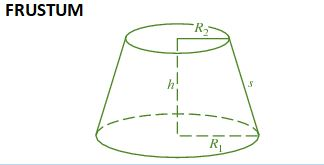Π(R1 + R2)s + (R12 + R22) Π(R1 + R2)s ⅓Πh(R12 + R22 + R1R2) √(h2 + (R1 – R2)2)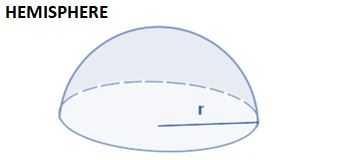3Πr2 2Πr2 2/3Πr3 No Slant height or diagonal

You might be acquainted with all the solids given in the above table and have done questions in your high school on them but now let’s learn about some more solids that you might be unaware of.

Prism

A Prism is a polyhedron comprising n-sided polygonal base congruent with a face parallel to it whose lateral faces are parallelogram and has a same cross section all along its length.

• Everyone must have heard the name Prism and might now a little about it through Physics or may be some of you have don’t remember anything at all. Today, we’ll tell you the geometric properties of Prism.
• The number of lateral faces is equal to number of sides in the polygonal base. Thus, the base could be triangular, quadrangular, hexagonal etc.
• A prism is said to be right, if the side edges are perpendicular to the ends. For example, cuboids and cubes.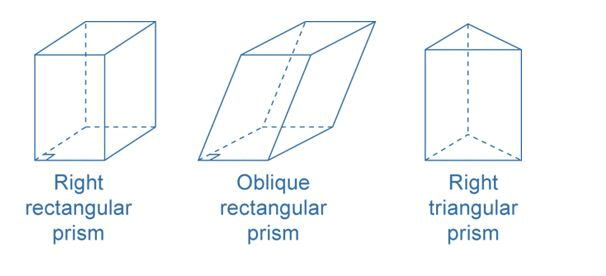Mensuration of Prism

• Curved Surface area of Prism: perimeter of the base x Height
• Total Surface area of Prism: Lateral surface area + (2 x base area)
• Volume of Prism: Base area x height

Pyramid

A pyramid is a polyhedron formed by connecting a polygonal base and a point called apex.  The lateral surface of pyramids form triangles.

• With the word pyramid what first pops up in our mind are the pyramids of Egypt. And you are right, the pyramid is of similar in structure with the pyramids of Egypt. But it's just that the pyramids of Egypt are one kind of pyramids with a square base.
• A pyramid with an n-sided base has n+1 vertices, n+1 faces, and 2n edges.
• A right pyramid has its apex directly above the centroid of its base.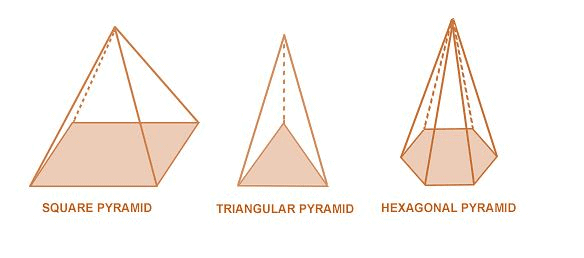Mensuration of Pyramid

• Curved surface area of Pyramid: perimeter/2 x slant height
• Total surface area of Pyramid: curved surface area + area of the base
• Volume of the Pyramid: Base area x ⅓ x height

Differences Between 2D and 3D shapes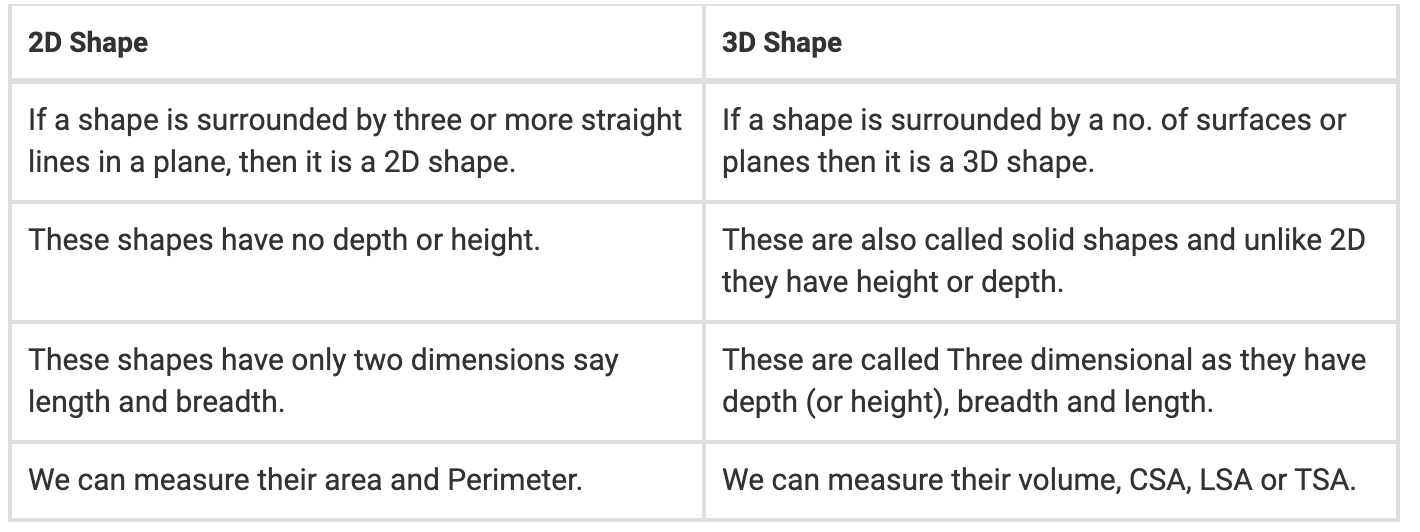Since now we are familiar with all the solids and the formulas to calculate their surface area and volume we can move on to try solving some questions but before moving forth to that keep these important points in your mind that will assist you in solving questions in simplified manner:

CAT Mensuration Shortcuts & Important Points:

• Use Diagrammatic representation/visualisation: As we are talking about 3D here, it’s crucial that we visualize the objects specified in the question. If we are unable to diagrams from the information given in the question then the whole exercise is futile. Thus, the pictorial representation of solid is the key piece in solving the question. If we are able to clearly define the interconnectedness between different solids half of the question is done. What left is to plug in the formulas using the given and interpreted parameters and you are done!
• Use Symmetry: Solids such as cube, spheres etc. are symmetric and thus questions can be solved with the help of symmetry. But to make use of symmetry make sure you understand the concept properly and know it’s application as well.
• Shortcuts & Formulas: If H is the height of the complete cone from which the frustum is cut, then from similar triangles
r/R = (H-h)/h
1. If a sphere is inscribed inside the cube of side a, then the radius of the sphere will be a/2. If the sphere is circumscribed about the cube of side a then the radius of sphere will be √3a/2.
2. If the largest possible sphere is inscribed in the cylinder of radius ‘R’ and height ‘h’, its radius r will be
r = h/2 (if 2R>h)
r = R (if 2R<h)
• Answering questions in percentage: So many times, you have a question in which you have to answer the percentage area wasted. In all those cases, you can avoid the actual values given and take the values, which are more suitable for calculation as it would not affect your answer in percentage.

CAT Mensuration Questions

Problem 1: If three cubes are placed adjacently in a row, then the ratio of the total surface area of the new cuboid to the sum of the surface area of the three cubes will be
(1) 1:3
(2) 2:3
(3) 5:9
(4) 7:9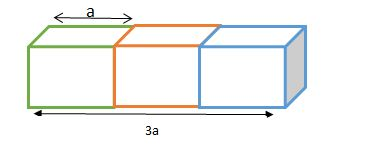We are given 3 cubes in the above question each with side a cm. When the cubes are placed adjacent in a row then the cuboid is formed with length 3a cm (a + a+ a) and height and breadth as a cm. Thus,
T.S.A of the cuboid = 2(3a*a + a*a + 3a*a)
= 14a2 cm2
T.S.A. of three cubes = 3*6a2
= 18a2 cm2
Thus, the ratio of T.S.A of cuboid to that of cube will be
14a2 cm2: 18a2 cm2
7 : 9

Try yourself:The surface area of cuboid-shaped box having length= 80 cm, breadth= 40cm and height= 20cm is:

Problem 2: X and Y are two cylinders of the same height. The base of the X has diameter that is half the diameter of the base of Y. If the height of X is doubled, the volume of X becomes
(1) Equal to the volume of Y
(2) Double the volume of Y
(3) Half the volume of Y
(4) Greater than the volume of Y

Original Solids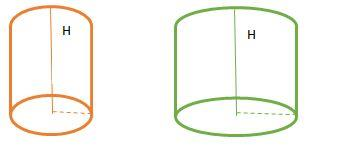There are two cylinders X and Y each with height H and diameter of X is 1/2 of the diameter of Y. Thus, volume of each solid is
Volume of X = Πr²H
Volume of Y = Π(2r)² H = 4ΠrH
Now, if the height of X is doubled then,
Volume of X = Πr²2H = 2 Πr²H = ½ x 4ΠrH
Thus, the resultant cylinder has volume has volume ½ of Y.

Try yourself:If the radius of a cylinder is 4cm and height is 10cm, then the total surface area of a cylinder is:

Problem 3: Water is poured into an empty cylindrical tank at a constant rate for 5 minutes. After the water has been poured into the tank, the depth of the water is 7 feet. The radius of the tank is 100 feet. Which of the following is the best approximation for the rate at which the water was poured into the tank
(1) 140 cubic feet/sec
(2) 440 cubic feet/sec
(3) 700 cubic feet/sec
(4) 2200 cubic feet/sec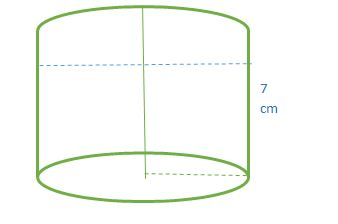We need to find out the rate at which water is flown in tank. The volume of water flown in tank in 5 minutes = Πr²h= (22/7 * 100² * 7)
=220000 cm²
The rate at which water is flowing in the tank is = (220000/5*60) = 733.33 ft./sec

Try yourself:The surface area of a sphere of radius 14 cm is:

Problem 4: A conical cavity is drilled in a circular cylinder of 15 cm height and 16 cm base diameter. The height and base diameter of the cone is same as those of cylinder. Determine total surface area of the remaining solid?
(1) 215 Π cm2
(2) 376 Π cm2
(3) 440 Π cm2
(4) 542 Π cm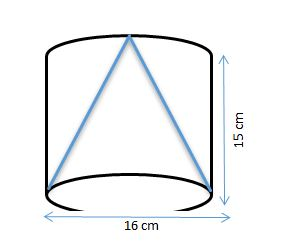In this question, we are given a cylinder out of which a cone is scraped out and we need to find out total surface area of the remaining solid given in the above figure.
Therefore, T.S.A of the remaining solid = C.S.A of cylinder + C.S.A of the cone + area of the base of cylinder
= 2Πrh + Πrl + Πr²
= Π(2*8*15 + 8*17 + 8²)
= Π(240 + 136 + 64)
= 440 cm²

Try yourself:The plastic paint in a Asian paint container is sufficient to paint an area equal to 93.75m2 How many blocks of dimensions 22.5 cm × 10 cm × 7.5 cm can be painted out of this container

Problem 5: Base of a right prism is a rectangle the ratio of whole length and breath is 3:2. If the height of the prism is 12 cm and total surface area is 288 cm2 then the volume of the prism is

(1) 291 cm3
(2) 288 cm3
(3) 290 cm3
(4) 286 cm3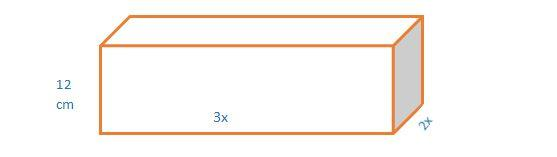Since, the prism has rectangular base then the prism formed is cuboid with the ratio of length and breath is 3:2 and height is 12 cm. Therefore T.S.A of cuboid = 288 cm²
288 = 2(lb + bh + hl)
288 =2(3x*2x + 2x*12+ 12*3x)
288 = 2(6x² + 24x + 36x)
144 = 6x² + 60x
x² + 10x – 24 = 0
x² + 12x – 2x – 24 = 0
(x + 12) (x – 2) = 0
x = 2
Therefore, length is 6 cm and breadth is 4 cm. Thus, volume of cuboid = lbh = 6 x 4 x 12 = 288 cm3

Try yourself:Find the maximum length of the rod that can be kept in a cuboidal box of sides 30 cm, 20 cm and 10 cm.

Offer running on EduRev: Apply code STAYHOME200 to get INR 200 off on our premium plan EduRev Infinity!

## Quantitative Aptitude (Quant)

116 videos|131 docs|131 tests

,

,

,

,

,

,

,

,

,

,

,

,

,

,

,

,

,

,

,

,

,

;